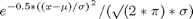## Loren on the Art of MATLABTurn ideas into MATLAB

Note

Loren on the Art of MATLAB has been archived and will not be updated.

# Trying to Match Behavior of a New Function to an Existing One

We might want to a write a function that mimics the behavior of another related function, specifically with respect to input shapes, etc. Ideally, we'd like to write it so we don't need to update our function if the related function changes how it works, again with respect to input shapes and sizes, for example.

### Error Checking

In a perfect world, we'd do enough error checking so users get a reasonable error message when appropriate. If the error conditions change for the original function we're mimicking, we would normally need to change our function as well to match the new behavior.

### Model our Function after the Arithmetic Operators

Suppose we want a function to behave like the arithmetic operators in MATLAB. Here's the relevant rule for plus, and it applies to all the elementwise operators and most of the elementwise functions in MATLAB:

• + Addition or unary plus. A+B adds A and B. A and B must have the same size, unless one is a scalar. A scalar can be added to a matrix of any size.

### The Function

We'd like to computeOften, when I am creating a new M-file, I often include the error checking at the top of the file. In the function I describe here, I have made an effort to generalize the error checking so it could be reused, not my usual starting point!

### Here's the code.

dbtype expMuSigChk
1     function y = expMuSigChk(x,mu,sigma)
2     % Exponential function with parameters mu and sigma.
3
4     % Check sizes.
5     if chkSz(x,mu,sigma) == 0
6         error('LSBlog:sizeMismatch','Non-scalar arguments must follow MATLAB dimension matching rules.');
7     end
8     % Since chkSz passed, we can now do our computations.
9     y = exp(-0.5 * ((x - mu)./sigma).^2) ./ (sqrt(2*pi) .* sigma);
10
11    function tf = chkSz(varargin)
12    % Collect info on nonscalarsizes and return false if they aren't all the
13    % same.
14    nonscalarsizes = {};
15    % convert sizes to strings so we can use unique on them
16    for k = 1:length(varargin)
17        if isscalar(varargin{k})
18            continue
19        end
20        nonscalarsizes{end+1} = int2str(size(varargin{k}));
21    end
22    if isempty(nonscalarsizes)
23        tf = true;
24    else
25        if length(unique(nonscalarsizes)) > 1
26            tf = false;
27        else
28            tf = true;
29        end
30    end
31



Notice that there are about 20 lines (including comments) for the error checking AND if MATLAB rules were to ever change, this check function here would have to change as well. It would be far too easy to forget about this and then be surprised when the behavior of expMuSigChk was no longer like MATLAB.

### Alternate Program

Instead we can write a program that takes advantage of MATLAB effectively doing the error checking for us. We try to do the calculation, and if it fails, we issue and error.

dbtype expMuSig
1     function y = expMuSig(x,mu,sigma)
2     %Exponential function with parameters mu and sigma.
3     try
4         y = exp(-0.5 * ((x - mu)./sigma).^2) ./ (sqrt(2*pi) .* sigma);
5     catch
6         error('LSBlog:sizeMismatch','Non-scalar arguments must follow MATLAB dimension matching rules.');
7     end